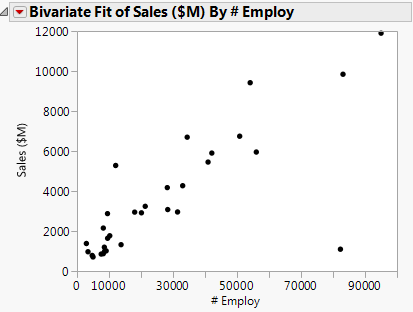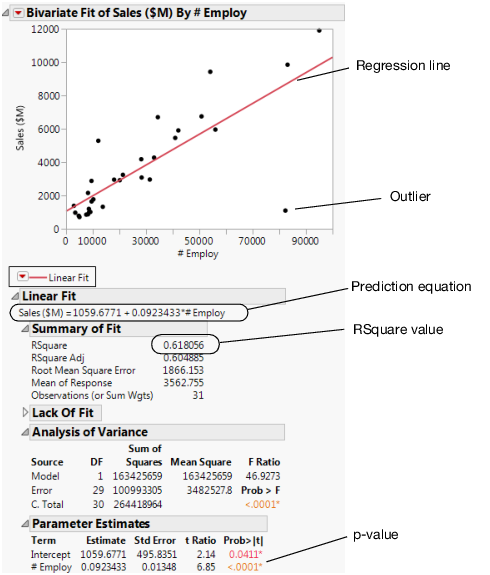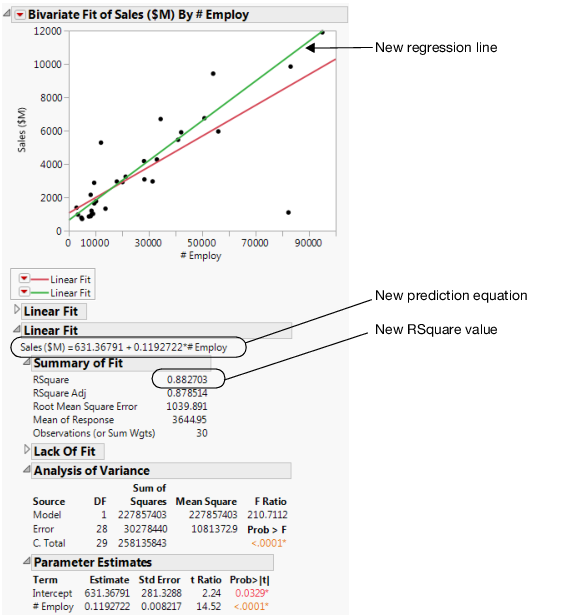Discovering JMP > Analyze Your Data > Analyze Relationships > Use Regression with One Predictor
Publication date: 08/13/2020

## Use Regression with One Predictor

### Scenario

This example uses the Companies.jmp data table, which contains financial data for 32 companies from the pharmaceutical and computer industries.

Intuitively, it makes sense that companies with more employees can generate more sales revenue than companies with fewer employees. A data analyst wants to predict the overall sales revenue for each company based on the number of employees.

To accomplish this task, do the following:

### Discover the Relationship

First, create a scatterplot to see the relationship between the number of employees and the amount of sales revenue. This scatterplot was created in Create the Scatterplot in the Visualize Your Data section. After hiding and excluding one outlier (a company with significantly more employees and higher sales), the plot in Figure 5.12 shows the result.

Figure 5.12 Scatterplot of Sales (\$M) versus # EmployThis scatterplot provides a clearer picture of the relationship between sales and the number of employees. As expected, the more employees a company has, the higher sales that it can generate. This visually confirms the data analyst’s guess, but it does not predict sales for a given number of employees.

### Fit the Regression Model

To predict the sales revenue from the number of employees, fit a regression model. Click the Bivariate Fit red triangle and select Fit Line. A regression line is added to the scatterplot and reports are added to the report window.

Figure 5.13 Regression LineWithin the reports, look at the following results:

the p-value of <.0001

the RSquare value of 0.618

From these results, the data analyst can conclude the following:

The p-value for the #Employ model term is small. This supports that at the 0.05 significance level the coefficient for #Employ is not zero. Therefore, including the number of employees in the prediction model significantly improves the ability to predict average sales over a model without the number of employees.

The RSquare value of 0.618 indicates that this model explains about 62% of the variability in sales. The RSquare value is the coefficient of determination and indicates the proportion of the variance in the dependent (response) variable that is explained by your model. RSquare can range from 0 to 1. A model with an RSquare of 0 has no explanatory power. A model with an RSquare of 1 predicts the response perfectly.

### Predict Average Sales

Use the regression model to predict the average sales a company might expect if they have a certain number of employees. The prediction equation for the model is included in the report:

Average sales = 1059.68 + 0.092*employees

For example, in a company with 70,000 employees sales are predicted to be about \$7,500:

\$7,499.68 = 1059.68 + 0.092*70,000

In the lower right area of the current scatterplot, there is an outlier that does not follow the general pattern of the other companies. The data analyst wants to know whether the prediction model changes when this outlier is excluded.

#### Exclude the Outlier

1. Click the outlier.

2. Select Rows > Exclude/Unexclude.

3. To fit this model, click red triangle next to Bivariate Fit of Sales (SM) By # Employ and select Fit Line.

The following are added to the report window (Figure 5.14):

a new regression line

a new Linear Fit report, which includes:

a new prediction equation

a new RSquare value

Figure 5.14 Comparing the Models### Interpret the Results

Using the results in Figure 5.14, the data analyst can make the following conclusions:

The outlier was pulling down the regression line for the larger companies, and pulling the line up for the smaller companies.

The new model for the data without the outlier is a stronger model than the first model. The new RSquare value of 0.88 is higher and closer to 1 than the initial analysis.

### Draw Conclusions

Using the new prediction equation, the predicted average sales for a company with 70,000 employees can be calculated as follows:

\$8961.37 = 631.37 + 0.119*70,000

The prediction from the first model was about \$7500. The second model predicts a sales total of about \$8960 or an increase of \$1460 as compared to the first model.

The second model, after removing the outlier, describes and predicts sales totals based on the number of employees better than the first model. The data analyst now has a good model to use.